2018

## Separating and predicting

### Discrimination

• Separating objects in an existing data set
• Find which variables that best separate the $$g$$ groups

### Classification

• Predict group membership for a new observation
• Allocate new objects to known groups

## Example 1: Iris DatasetThis famous (Fisher’s or Anderson’s) iris data set gives the measurements in centimeters of the variables:

• Sepal Length (X1)
• Sepal Width (X2)
• Petal Length (X3)
• Sepal Width (X4)

## Example 1: Iris Dataset

load("_data/iris.train.Rdata")
head(iris.train)
   Sepal.Length Sepal.Width Petal.Length Petal.Width    Species
61          5.0         2.0          3.5         1.0 versicolor
62          5.9         3.0          4.2         1.5 versicolor
63          6.0         2.2          4.0         1.0 versicolor
64          6.1         2.9          4.7         1.4 versicolor
65          5.6         2.9          3.6         1.3 versicolor
66          6.7         3.1          4.4         1.4 versicolor
• Can we classify versicolor or virginica based on X-variables?
• What’s the best method?

## Iris Dataset: Pairs plot

pairs(iris.train[, -5], bg = iris.train[, "Species"], pch = 21)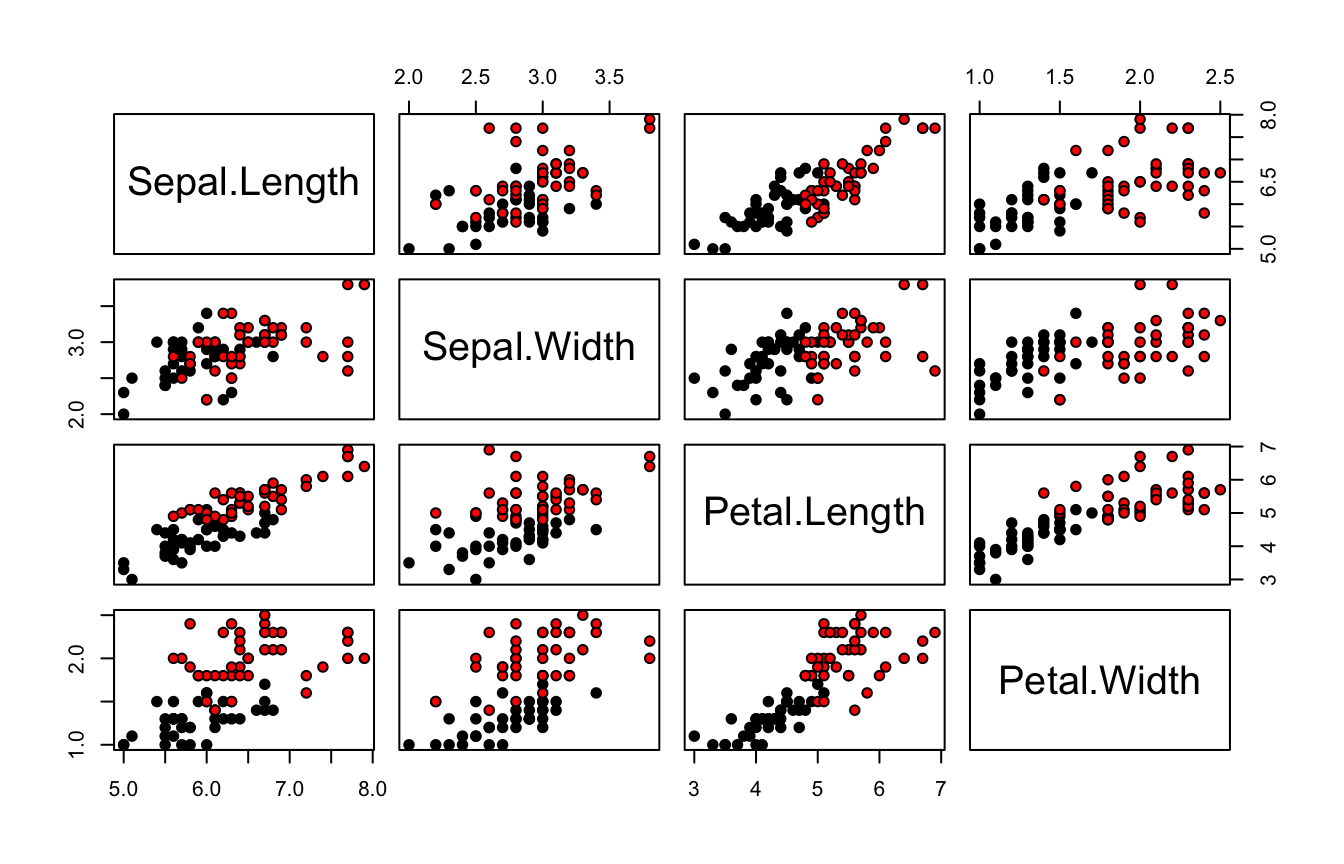## Example 2: Lizards

load("_data/Lizard.Rdata")
pairs(Lizard[, -4], bg = Lizard$Sex, pch = 21, cex = 1.5)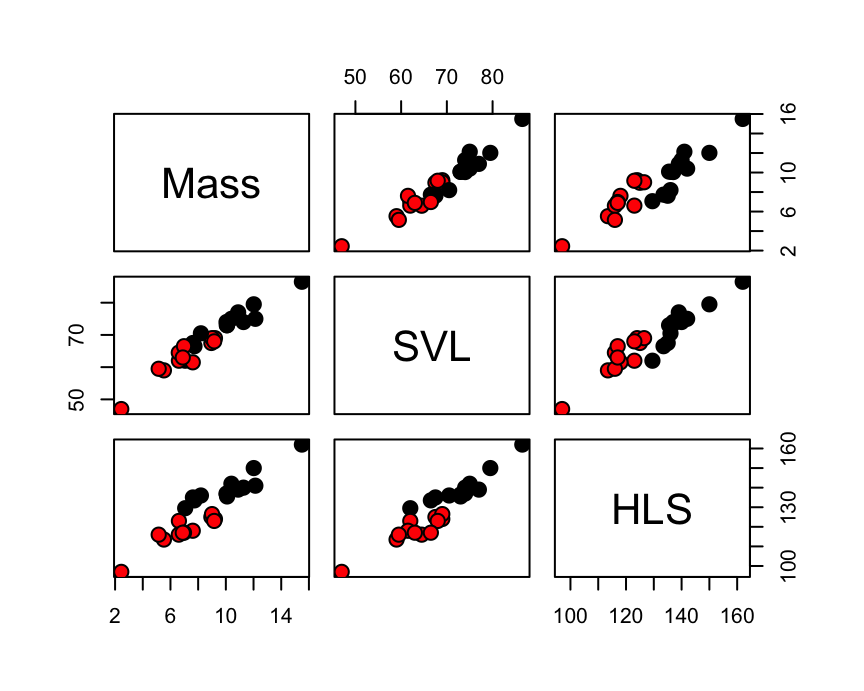variable Description Mass Weight of Lizard (in grams) SVL Snout-vent length (in mm) HLS Hind limb span (in mm) • In addition to sex we have measured $$k = 3$$ more variables on lizards as above. • Can we discriminate Sex on the basis of the body measures? ## Example 2: Lizards You must enable Javascript to view this page properly. ### Lizard Dataset head(Lizard)  Mass SVL HLS Sex 1 5.526 59.0 113.5 f 2 10.401 75.0 142.0 m 3 9.213 69.0 124.0 f 4 8.953 67.5 125.0 f 5 7.063 62.0 129.5 m 6 6.610 62.0 123.0 f ## Finding decision regions In descriminant analysis we search for decision regions for the $$g$$ known groups (classes). Regions $$R_1, R_2, \ldots, R_g$$ ### Univariate Descrimination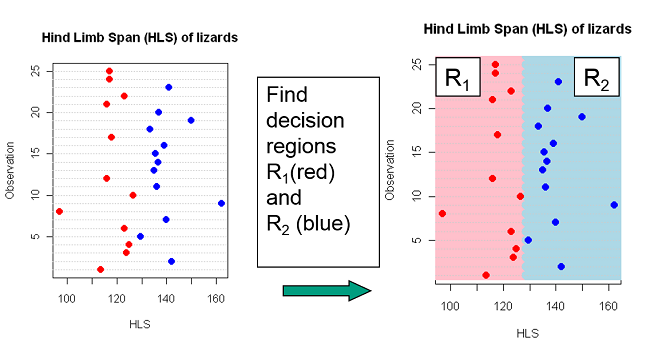### Bivariate discrimination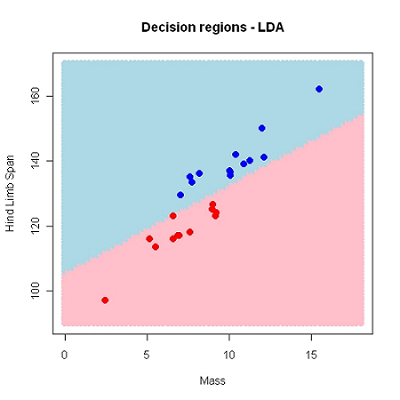Using HLS and Mass as predictors $$(k=2)$$, we have used a linear decision border. This may also be non-linear. ## Finding decision regions You must enable Javascript to view this page properly. ### Tri-variate discrimination • Using all variables $$(k=3)$$, • A linear discriminator is a flat plane in the 3D-space ## Most probable group • Perfect discrimination/classification may not be possible. • The idea is to find decision regions $$R_1, R_2, \ldots, R_g$$ that minimizes the probability of doing misclassification. • Intuitively we would allocate an observation to the group which is most probable given the observed value of the predictors • If, for example, the HLS of a new lizard is 130, it seems most probable based on previous data that the lizard is a male. ## Bayes rule Let $$P(c_i|x_1,\ldots, x_p)$$ be the probability of class $$i$$ given the observed variables. Then by Bayes rule: $P(c_i|x_1,\ldots, x_p) \propto f(x_1,\ldots, x_p|c_i)p_i$ where $$p_i$$ is the a priori probability of observing an object from class $$c_i$$ and… $$f(x_1,\ldots, x_p|c_i)$$ is the likelihood (“probability”) of the observed data given class $$i$$. ## A simple classification scheme Allocate a new observation with observed variables $$x_1^*, x_2^*, \ldots x_p^*$$ to the class which maximizes $f(x_1^*,\ldots, x_p^*)p_i$ We need to estimate $$f(x_1^*,\ldots, x_p^*)$$ from the training data and determine $$p_i$$. In linear discriminant analysis (LDA) we assume that $$f(x_1^*,\ldots, x_p^*)$$ is a normal distribution, hence we must estimate the mean and (a common) variance for observations from each class. Often we assume uniform prior: $$p_1 = p_2 = \cdots = p_g = 1/g$$ . ## Lizards example Assume equal probabilities for females ($$c_1$$) and males ($$c_2$$) a priori: $$p_1 = p_2 = 0.5$$ Assume $$x_1$$ = HLS is the only predictor. From the data we estimate $$\mu_1$$, $$\mu_2$$ and common $$\sigma^2$$ for both classes. Let $$f_1$$ and $$f_2$$ denote these two normal distributions. Classification rule: Classify a new lizard as female if $f_1(x_1^*)p_1 \geq f_2(x_1^*)p_2$ or, alternatively if $\frac{f_1(x_1^*)p_1}{f_2(x_1^*)p_2}\geq 1$ ## Visual representation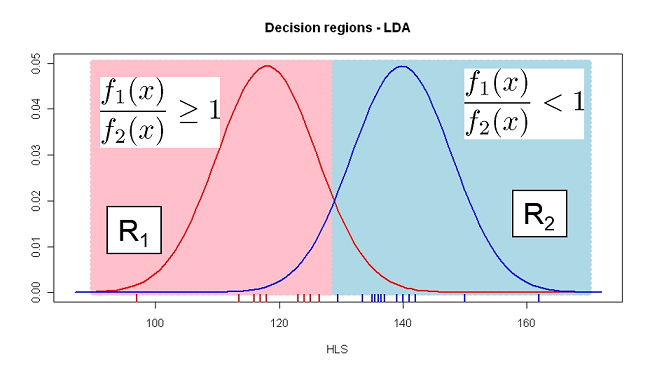## Linear Discreminent Analysis (LDA) in R The fit is presented as a confusion matrix and accuracy of classification library(MASS) DAModel.1 <- lda( formula = Sex ~ HLS, data = Lizard, prior = rep(0.5, 2)) pred_mdl <- predict(DAModel.1)  Mass SVL HLS Sex 1 5.526 59.0 113.5 f 2 10.401 75.0 142.0 m 3 9.213 69.0 124.0 f 4 8.953 67.5 125.0 f 5 7.063 62.0 129.5 m 6 6.610 62.0 123.0 f R also returns the predicted classes (fitted) and $$P(c_i|x_1^*)$$ also known as the posterior probability. head(pred_mdl$posterior, 4)
           m          f
1 0.00590689 0.99409311
2 0.98750273 0.01249727
3 0.16418501 0.83581499
4 0.21513565 0.78486435
head(pred_mdl$class)  f m f f m f Levels: m f ## Confusion Matrix and classification error From the observed and predicted class of category Sex, we can construct a confusion matrix and calculate classification error. Remember the classification rule is, $f_1(x_1^*)p_1 \geq f_2(x_1^*)p_2$ ### Can you answer? • Can it classify with same accuracy for new observation? • Since this also depends on prior probability, how will it classify with different priors. ### Confusion Matrix confusion(Lizard$Sex, pred_mdl$class)  True Predicted m f m 13 0 f 0 12 Total 13 12 Correct 13 12 Proportion 1 1 ### Accuracy N correct/N total = 25/25 = 1 ## Using different priors If we assume males are more abundant: $$p_1 = 0.2$$ and $$p_2=0.8$$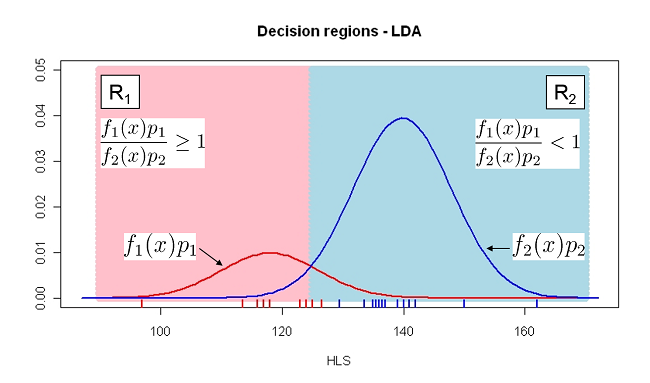## LDA in R using different priors DAModel.2 <- lda( formula = Sex ~ HLS, data = Lizard, prior = c(0.8,0.2)) pred_mdl <- predict(DAModel.2) confusion(Lizard$Sex, pred_mdl$class)  True Predicted m f m 13 2.0000000 f 0 10.0000000 Total 13 12.0000000 Correct 13 10.0000000 Proportion 1 0.8333333 N correct/N total = 23/25 = 0.92 ### The default choice prior In R the default choice of prior is “empirical” which means that the priors are set equal to the group proportions in the data set Hence, if there are $$n_i$$ observations in group $$i$$ and $$N = \sum_{i=1}^g n_i$$ is the total observation number, then $\hat{p}_i = \frac{n_i}{N}$ This may be reasonable if one assumes that the sample sizes reflect population sizes. ## Bivariate LDA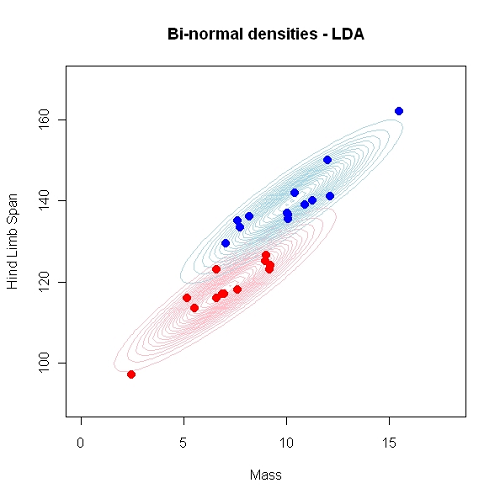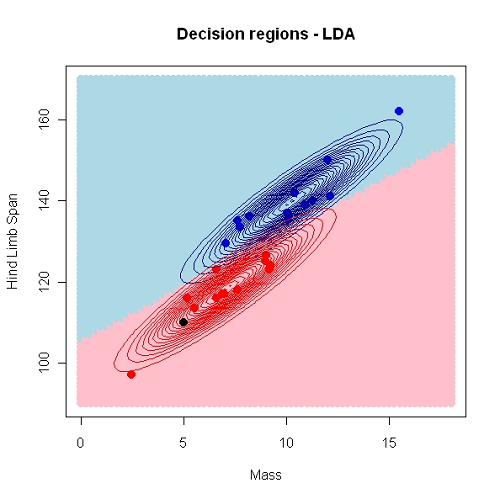## Quadratic discriminant analysis (QDA) ### A Bivariate QDA - Decision regions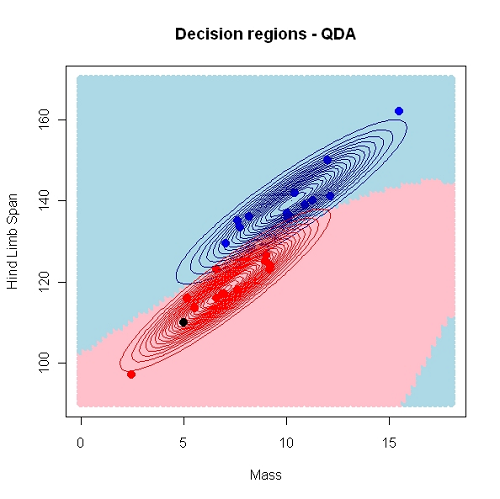QDA differs from LDA in two ways: 1. Class specific variances 2. Non-linear decision borders library(MASS) QDA_model <- qda( formula = Sex ~ HLS, data = Lizard, prior = c(0.8,0.2)) pred_mdl <- predict(QDA_model) ## Model selection/evaluation • We often want to compare the performance of different classifiers. • Many available statistics for this purpose, but we will consider the accuracy or the apparent error rate (APER) • The performance of a classifier may as we’ve seen be summarized in a confusion matrix • From the error rate, we can also compute the classification accuracy ### Apparent Error Rate (APER) Consider the confusion matrix as, True Male Female Predicted Male $$n_m/m$$ $$n_m/f$$ Female $$n_f/m$$ $$n_f/f$$ Total $$n_m$$ $$n_f$$ $\text{APER} = \frac{n_m/f + n_f/m}{n_m + n_f} = \frac{\text{Total Incorrect}}{\text{Total Observation}}$ Classification accuracy will be $$1 - \text{APER}$$. ## Example with R: SVL as predictor DAModel.4 <- lda( formula = Sex ~ SVL, data = Lizard, prior = rep(0.5, 2)) pred_mdl <- predict(DAModel.4) confusion(Lizard$Sex, pred_mdlclass)  True Predicted m f m 10.000 2.000 f 3.000 10.000 Total 13.000 12.000 Correct 10.000 10.000 Proportion 0.769 0.833 ### Probabilities of misclassification - APER \begin{aligned} P(\texttt{female | male}) &= \dfrac{3}{13} = 0.231 \\ P(\texttt{male | female}) &= \dfrac{2}{12} = 0.167 \end{aligned} $\text{Apparent error rate (APER)} = \frac{3 + 2}{25} = 0.2$ Accuracy = $$1-\text{APER} = 0.8$$ ## Validation (Test-data or CV)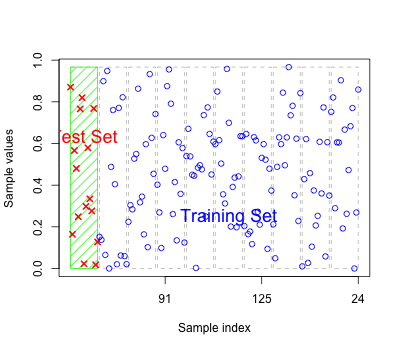• As for regression models the errors based on fitted values may reflect over-fitting • Should have new test data to predict to get an honest classification error. • Alternatively we can perform cross-validation ### Types of Cross-Validation • K-fold cross-validation and • Leave-one-out (LOO) Cross-validation ## LOO cross-validation: LDA with SVL as predictor da_model <- lda( formula = Sex ~ SVL, data = Lizard, prior = rep(0.5,2), CV = TRUE) confusion(LizardSex, da_model\$class)
            True
Predicted         m     f
m          10.000  3.00
f           3.000  9.00
Total      13.000 12.00
Correct    10.000  9.00
Proportion  0.769  0.75

Typically, as seen here, the accuracy goes down, and the APER increases when new samples are classified.

### APER (Error rate)

N incorrect/N total = 6/25 = 0.24

### Accuracy

N correct/N total = 19/25 = 0.76